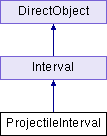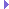ProjectileInterval Class Reference
Inheritance diagram for ProjectileInterval:## Public Member Functions

def __init__ (self, node, startPos=None, endPos=None, duration=None, startVel=None, endZ=None, wayPoint=None, timeToWayPoint=None, gravityMult=None, name=None, collNode=None)

def privInitialize (self, t)

def privInstant (self)

def privStep (self, t)

def testTrajectory (self)Public Member Functions inherited from Interval
def __init__ (self, name, duration, openEnded=1)

def __repr__ (self, indent=0)

def clearToInitial (self)

def finish (self)

def getDoneEvent (self)

def getDuration (self)

def getLoop (self)

def getName (self)

def getOpenEnded (self)

def getPlayRate (self)

def getState (self)

def getT (self)

def intervalDone (self)

def isPaused (self)

def isPlaying (self)

def isStopped (self)

def loop (self, startT=0.0, endT=-1.0, playRate=1.0)

def pause (self)

def popupControls (self, tl=None)

def privDoEvent (self, t, event)

def privFinalize (self)

def privInterrupt (self)

def privPostEvent (self)

def privReverseFinalize (self)

def privReverseInitialize (self, t)

def privReverseInstant (self)

def resume (self, startT=None)

def resumeUntil (self, endT)

def setDoneEvent (self, event)

def setLoop (self, loop=1)

def setPlayRate (self, playRate)

def setT (self, t)

def setupPlay (self, startT, endT, playRate, doLoop)

def setupResume (self)

def start (self, startT=0.0, endT=-1.0, playRate=1.0)

def stepPlay (self)Public Member Functions inherited from DirectObject
def __init__ (self)

def accept (self, event, method, extraArgs=[])

def acceptOnce (self, event, method, extraArgs=[])

def addTask (self, *args, **kwargs)

def detectLeaks (self)

def doMethodLater (self, *args, **kwargs)

def getAllAccepting (self)

def ignore (self, event)

def ignoreAll (self)

def isAccepting (self, event)

def isIgnoring (self, event)

def removeAllTasks (self)

def removeTask (self, taskOrName)

## Public Attributes

collNode

duration

endPos

implicitStartPos

node

parabola

startPos

startVel

trajectoryArgs

zAccPublic Attributes inherited from Interval
currT

doneEvent

duration

es

name

openEnded

pname

pstats

setTHooks

state

## Static Public Attributes

int gravity = 32.

notify = directNotify.newCategory('ProjectileInterval')

int projectileIntervalNum = 1Static Public Attributes inherited from Interval
notify = directNotify.newCategory("Interval")

int playbackCounter = 0Static Public Attributes inherited from DirectObject
def accept_once = acceptOnce

def add_task = addTask

def detect_leaks = detectLeaks

def do_method_later = doMethodLater

def get_all_accepting = getAllAccepting

def ignore_all = ignoreAll

def is_accepting = isAccepting

def is_ignoring = isIgnoring

def remove_all_tasks = removeAllTasks

def remove_task = removeTask

## Additional Inherited MembersProperties inherited from Interval
done_event = property(getDoneEvent, setDoneEvent)

open_ended = property(getOpenEnded)

play_rate = property(getPlayRate, setPlayRate)

stopped = property(isStopped)

t = property(getT, setT)

## Detailed Description

```ProjectileInterval class: moves a nodepath through the trajectory
of a projectile under the influence of gravity```

## ◆ __init__()

 def __init__ ( self, node, startPos = `None`, endPos = `None`, duration = `None`, startVel = `None`, endZ = `None`, wayPoint = `None`, timeToWayPoint = `None`, gravityMult = `None`, name = `None`, collNode = `None` )
```You may specify several different sets of input parameters.
(If startPos is not provided, it will be obtained from the node's
position at the time that the interval is first started. Note that
in this case you must provide a duration of some kind.)

# go from startPos to endPos in duration seconds
startPos, endPos, duration
# given a starting velocity, go for a specific time period
startPos, startVel, duration
# given a starting velocity, go until you hit a given Z plane
startPos, startVel, endZ
# pass through wayPoint at time 'timeToWayPoint'. Go until
# you hit a given Z plane
startPos, wayPoint, timeToWayPoint, endZ

You may alter gravity by providing a multiplier in 'gravityMult'.
'2.' will make gravity twice as strong, '.5' half as strong.
'-1.' will reverse gravity

If collNode is not None, it should be an empty CollisionNode
which will be filled with an appropriate CollisionParabola
when the interval starts.  This CollisionParabola will be set
to match the interval's parabola, and its t1, t2 values will
be updated automatically as the interval plays.  It will *not*
be automatically removed from the node when the interval
finishes.```

## ◆ privInitialize()

 def privInitialize ( self, t )

Reimplemented from Interval.

## ◆ privInstant()

 def privInstant ( self )

Reimplemented from Interval.

## ◆ privStep()

 def privStep ( self, t )

Reimplemented from Interval.

## ◆ testTrajectory()

 def testTrajectory ( self )

## ◆ collNode

 collNode

## ◆ duration

 duration

 endPos

## ◆ gravity

 int gravity = 32.
static

## ◆ implicitStartPos

 implicitStartPos

 node

## ◆ notify

 notify = directNotify.newCategory('ProjectileInterval')
static

## ◆ parabola

 parabola

## ◆ projectileIntervalNum

 int projectileIntervalNum = 1
static

## ◆ startPos

 startPos

## ◆ startVel

 startVel

## ◆ trajectoryArgs

 trajectoryArgs

 zAcc